Open in App
Not now

# Loops in LISP

• Last Updated : 06 Oct, 2021

Loops allow executing a set of instructions repeatedly while some condition is true. LISP provides the following types of loops:

### 1. dotimes loop:

The dotimes loop allows executing instructions for a fixed number of times.
Syntax:

```(dotimes( variableName numberOfIterations ) (
expressions
))```

Where,

• variableName => Name of the variable, this can be used to get current iteration number
• numberOfIterations => Total number of iterations
• expressions => Statements to be executed in each iteration

Example:

## Lisp

 `(``dotimes` `(a ``4``)``  ``(print a))``(write``-``line "")`

Output: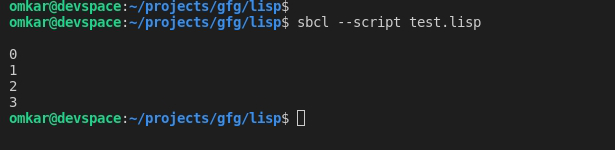You can also return from the loop if a certain condition is met

## Lisp

 `(``dotimes` `(i ``7``)``   ``(``if` `(> i ``5``)``       ``(return)``       ``(print i))``    ``)``(write``-``line "")`

Here, the loop is set to iterate 7 times, however, when the value becomes more than 5, the loop stops because the condition is met.
Output: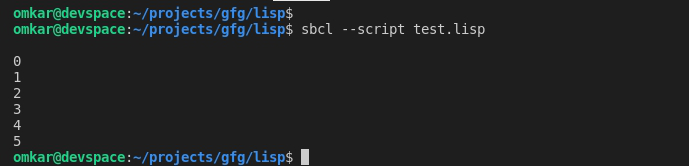### 2.  loop:

The loop construct allows executing some statement(s) repeatedly until it finds a return statement.

Syntax:

```( loop
(expressions)
( when (condition) (return returnValue))
)```

Where,

• expressions => Statements to be executed in each iteration
• condition => Condition that specifies when to exit loop
• returnValue => Value to be returned

Example:

## Lisp

 `(``defvar` `n ``3``)``(``loop``    ``(print n)``    ``(setq n (``+` `n ``1``))``    ``(``when` `(> n ``6``) (return n))``)``(write``-``line "")`

Here, n is printed and incremented until it becomes greater than 6.
Output: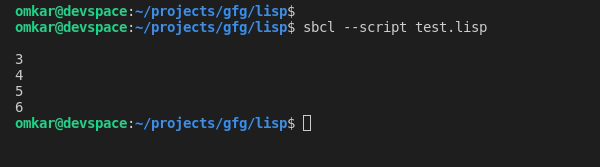### 3. loop for:

The loop for construct is similar to the for-loops in popular languages like java, c++, etc. It can also be used to traverse lists and other data structures.

Syntax:

```( loop for variableName from startValue to endValue by changeValue do
(expressions)
)```

Where,

• variableName => Name of the variable, this can be used to get the value of variable in current iteration.
• startValue => Initial value of variable
• endValue => End value of variable (after last iteration)
• changeValue => Specifies by how much to increment or decrement value after every iteration
• expressions => Statements to be executed in each iteration

Example:

## Lisp

 `; initialize x``=``12``, execute instructions, ``; increment it by ``3` `and perform iteration until it is less than ``25``(``loop` `for x from ``12` `to ``25` `by ``3` `do``    ``(print x)``)``(write``-``line "")`

Output: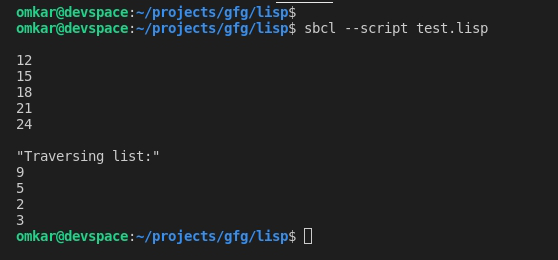### 4. do:

The do construct allows a structured form of iteration.
Syntax:

```(do ((variable_1    value_1   updated-value_1)
(variable_2   value_2   updated-value_2)
(variable_3   value_3   updated-value_3)
...)
(test return_value)
(s-expressions)
)```

Where,

• variable_1 => First variable
• value_1 => Initial Value for first variable
• updated-value_1 => Specifies how to update variable_1 after each iteration.
• variable_2 => Second variable
• value_2 => Initial Value for second variable
• updated-value_2 => Specifies how to update variable_2 after each iteration. … similar till variable_n
• test => Condition that specifies when to stop the loop
• return value => Value that is returned at the end of iteration
• expressions => Other statements to be executed in every iteration

The initial values of each variable are evaluated and bound to the respective variable. The updated value in every clause is with respect to an optional update statement which indicates how to update variables in each iteration.
The test is performed on each iteration and if the test expression results in a non-null or true value, the returned value is evaluated and returned.
If the last optional s-expression(s) are specified, they’re executed on every iteration while test expression results are true.
Example:

## Lisp

 `(do ((a ``0` `(``+` `2` `a))``   ``(b ``20` `( ``-` `b ``2``)))``   ``((``=` `a b)(``-` `a b))``   ``(``format` `t` `"~% a = ~d  b = ~d"` `a b)``)``(write``-``line "")`

Output: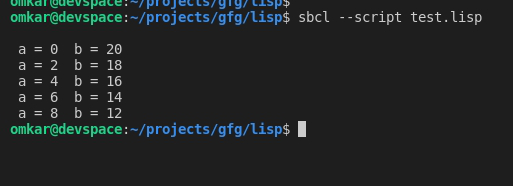### 5. dolist:

The dolist construct provides an easy way to traverse lists.

Syntax:

```(dolist (listItemVariable list)
(expressions)
) ```

Where,

• listItemVariable => Holds value of each list item during every iteration
• list => List to traverse
• expressions => Statements to be executed in every iteration

Example:

## Lisp

 `(``defvar` `nums `(``9` `5` `2` `3``))``(``dolist` `(item nums)``  ``(print item)``)``(write``-``line "")`

Output: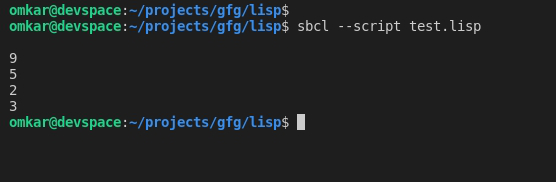References:

My Personal Notes arrow_drop_up